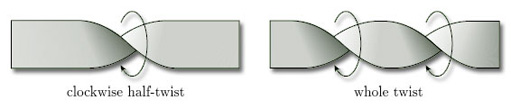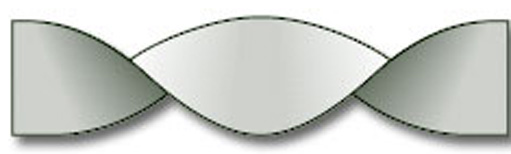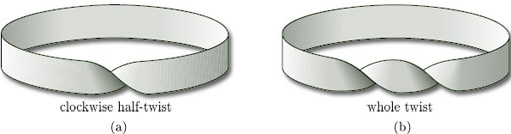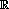Surfaces

Start this free course now. Just create an account and sign in. Enrol and complete the course for a free statement of participation or digital badge if available.

Free course

# 3 The orientability of surfaces

## 3.1 Surfaces with twists

In Section 3 we study the orientability of surfaces from an informal point of view. In particular, we take a detailed look at the projective plane and its properties. We start by examining some surfaces that resemble a Möbius band.

A cylinder or a Möbius band can be formed by gluing together the ends of a rectangular strip or band of paper either with or without twisting the paper before gluing. Does adding further twists to the band before gluing provide any more examples of surfaces?

We say that the band shown on the left of Figure 47 has a half-twist – and going from left to right it is a clockwise half-twist – while the band on the right has two clockwise half-twists, making a whole twist. A half-twist is throughradians; a whole twist is through 2radians.Figure 47

Clearly, the twists must be in the same direction (clockwise or anticlockwise), or they will cancel each other out, as Figure 48 shows.Figure 48 Two half-twists that cancel out

If we join up the ends of a band with a half-twist, we get a Möbius band, as shown in Figure 49(a). But what do we get if we join up the ends of a band with a whole twist, as in Figure 49(b)?Figure 49

To answer this question, we cut the band, as shown in Figure 50. We can then undo the whole twist, glue the cut edges back together (making sure that the two edges are in the same direction, as before), and obtain a cylinder.

This cutting and gluing process is an example of a homeomorphism, as we saw in Section 2.4. Thus:

a band with a whole twist is homeomorphic to a band with no twists.

Clearly, using the method outlined above, we can undo any number of whole twists, so that a band with any number of whole twists is homeomorphic to a band with no twists: a cylinder. However, we cannot undo a half-twist. Thus, for example, if we have a band with three whole twists and an extra half-twist, then we can untwist the three whole twists but we cannot do anything with the half-twist, so we have a Möbius band.

We can conclude that, topologically, there are just two types of band:

• bands with an even number of half-twists, which are homeomorphic to a cylinder;

• bands with an odd number of half-twists, which are homeomorphic to a Möbius band.

The intuitive difference we feel between a band with a number of twists and a band with no twists (cylinder) or one twist (Möbius band) is captured by a theorem which states that the homeomorphisms between such bands cannot be extended to homeomorphisms of3.

This categorisation of bands will prove useful when we discuss orientability in Section 3.2.

### Problem 11

Write down the boundary number β of a band with:

(a) three half-twists; (b) four half-twists.

(a) β = 1; (b) β = 2.

M338_1